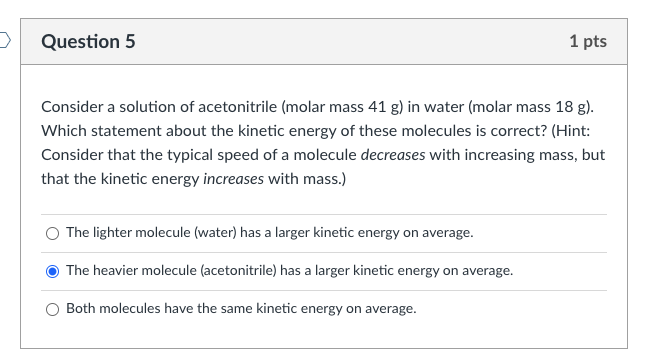Home / Expert Answers / Chemistry / consider-a-solution-of-acetonitrile-molar-mass-41-mathrm-g-in-water-molar-mass-18-pa597

# (Solved): Consider a solution of acetonitrile (molar mass $$41 \mathrm{~g}$$ ) in water (molar mass $$18 ...Consider a solution of acetonitrile (molar mass \( 41 \mathrm{~g}$$ ) in water (molar mass $$18 \mathrm{~g}$$ ). Which statement about the kinetic energy of these molecules is correct? (Hint: Consider that the typical speed of a molecule decreases with increasing mass, but that the kinetic energy increases with mass.) The lighter molecule (water) has a larger kinetic energy on average. The heavier molecule (acetonitrile) has a larger kinetic energy on average. Both molecules have the same kinetic energy on average.

We have an Answer from Expert